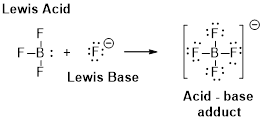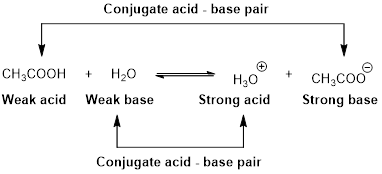Chapter 16, Problem 78PS

Chapter
Section
Textbook Problem

Which should be the stronger Brønsted acid, [V(H2O)6]2+ or [V(H2O)6]3+

Interpretation Introduction

Interpretation:

Stronger Bronsted acid has to be identified from the given compounds.

[V(H2O)6]2+ or [V(H2O)6]3+

Concept introduction:

Arrhenius Acids and Bases

Acid release hydrogen ion in water

Base release hydroxide ions in water.

HCl(aq) H+(aq) + Cl-(aq).........AcidNaOH(aq) Na+(aq)+ OH-(aq)...........Base

An acid is a substance that produces hydronium ions, H3O+ when dissolved in water.

Bronsted –Lowry definitions:

A Bronsted –Lowry acid is a proton donor, it donates a hydrogen ion, H+

A Bronsted-Lowry base is a proton acceptor, it accepts a hydrogen ion H+.Lewis definition:

A Lewis acid is a substance that can accept and share an electron pair.

A Lewis base is a substance that can donate and share an electron pair.Bronsted –Lowry conjugate acid-Base pairs:

When an acid is dissolved in water, the acid (HA) donates a proton to water to form a new acid (conjugate acid) and a new base (conjugate base).Explanation

The given complexes are [V(H2O)6]2+ and [V(H2O)6]3+

Let’s write the ionization reaction of each complex.

[V(H2O)6]2+(aq) +H2O(l) [V(H2O)5OH]+ (aq) + H3O+(aq)

+2 oxidation of the complex indicates that vanadium lose their two electrons

Still sussing out bartleby?

Check out a sample textbook solution.

See a sample solution

The Solution to Your Study Problems

Bartleby provides explanations to thousands of textbook problems written by our experts, many with advanced degrees!

Get Started

Find more solutions based on key concepts